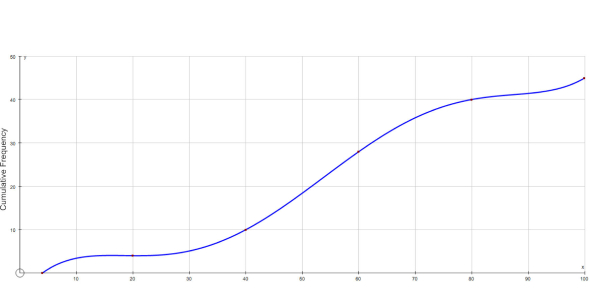# Cumulative Review Chapters 1 & 2

9 Questions | Attempts: 81SettingsThis is a quiz to see how much you remember from what we have talked about so far in math this year.

• 1.
Find the next three terms in the number pattern.0, 3, 7, 12, ____, ____, ____
• A.

18, 24, 32

• B.

18, 25, 32

• C.

17, 23, 30

• D.

18, 25, 33

• 2.
Evaluate. 4 x (3+7)
• A.

19

• B.

43

• C.

14

• D.

40

• 3.
Of twenty-eight students, four read both The Red Pony and The Hobbit.  Fifteen read the first book, but not the second.  Five students read neither book.  How many read only The Hobbit?
• A.

8 students

• B.

5 students

• C.

4 students

• D.

7 students

• 4.
Which word phrase best describes the expression x + y?
• A.

A number plus itself

• B.

Twice a number

• C.

A number more than two

• D.

A number plus another number

• 5.
Use mental math to solve k - 4 = 9.
• A.

5

• B.

9

• C.

13

• D.

36

• 6.
In which equation is the value of y the same as in the equation y + 19 = 39.
• A.

39 - y = 20

• B.

Y + 20 = 39

• C.

39 - 19 = y

• D.

Y x 19 = 39

• 7.
Which is the value of 64 - 4 x (2 + 13)?
• A.

4

• B.

34

• C.

47

• D.

900

• 8.
Use mental math to evaluate 4x - 9 for x = 6.
• A.

14

• B.

15

• C.

33

• D.

37

• 9.
Janet paid x for a DVD.  She gave the clerk a \$20 bill.  She received \$7 in change.  Which equation would you use to find the cost of the DVD?
• A.

7x = 20

• B.

20 = x + 7

• C.

X - 20 = 7

• D.

X + 20 = 7

## Related TopicsBack to top
×

Wait!
Here's an interesting quiz for you.